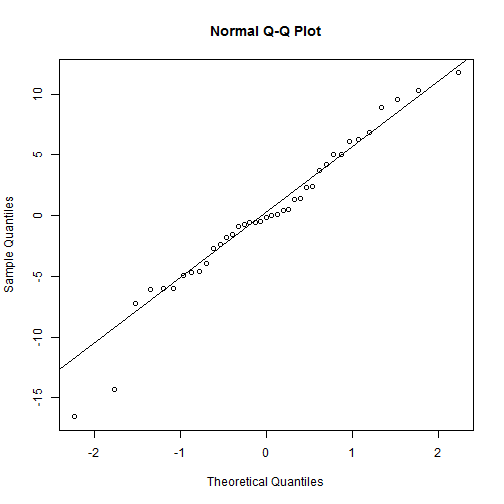# MASS Introductory Session

This is an R Markdown document. Markdown is a simple formatting syntax for authoring web pages (click the MD toolbar button in R Studio for help on Markdown).

When you click the Knit HTML button a web page will be generated that includes both content as well as the output of any embedded R code chunks within the document.

``````# A command to make MASS datasets available.
library(MASS)

# generate 1000 pairs of normal variates
x <- rnorm(1000)
y <- rnorm(1000)

# Histogram of a mixture of normal distributions.
truehist(c(x, y + 3), nbins = 25)
``````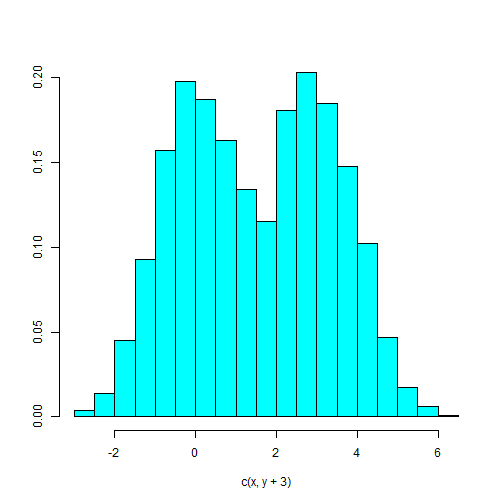``````
# 2D density plot
contour(dd <- kde2d(x, y))
``````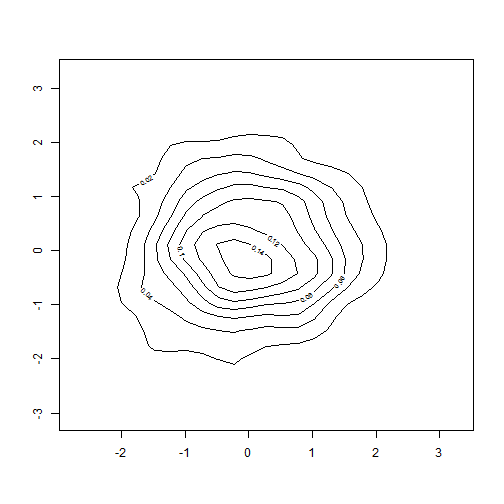``````
# Pseudo-color plot
image(dd)
``````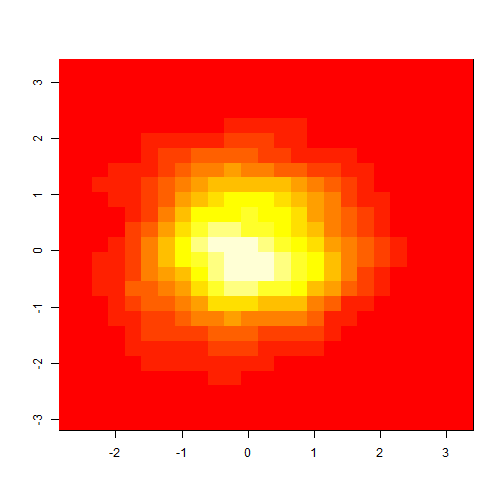``````# Make x = (1,1.5,2,...,19.5,20) and list it
x <- seq(1, 20, 0.5)
x
``````
``````##    1.0  1.5  2.0  2.5  3.0  3.5  4.0  4.5  5.0  5.5  6.0  6.5  7.0  7.5
##   8.0  8.5  9.0  9.5 10.0 10.5 11.0 11.5 12.0 12.5 13.0 13.5 14.0 14.5
##  15.0 15.5 16.0 16.5 17.0 17.5 18.0 18.5 19.0 19.5 20.0
``````
``````
# w will be used as a 'weight' vector and to give the standard deviations
# of the errors.
w <- 1 + x/2
y <- x + w * rnorm(x)

# Make a data frame of the three columns named x, y, and w and look at it.
# Remove the original x, y and w.
dum <- data.frame(x, y, w)
dum
``````
``````##       x       y     w
## 1   1.0  1.0084  1.50
## 2   1.5  4.3779  1.75
## 3   2.0  0.9178  2.00
## 4   2.5  2.4718  2.25
## 5   3.0  7.5329  2.50
## 6   3.5  2.9086  2.75
## 7   4.0  4.0597  3.00
## 8   4.5  7.1072  3.25
## 9   5.0  2.7417  3.50
## 10  5.5  5.9513  3.75
## 11  6.0 -0.1003  4.00
## 12  6.5 10.1198  4.25
## 13  7.0  0.8725  4.50
## 14  7.5  6.7014  4.75
## 15  8.0  3.7657  5.00
## 16  8.5  8.2129  5.25
## 17  9.0 13.6275  5.50
## 18  9.5  1.7811  5.75
## 19 10.0  9.9657  6.00
## 20 10.5 11.2661  6.25
## 21 11.0  5.4617  6.50
## 22 11.5 16.8678  6.75
## 23 12.0 18.0417  7.00
## 24 12.5 13.0930  7.25
## 25 13.0  7.3773  7.50
## 26 13.5 11.8460  7.75
## 27 14.0 11.1900  8.00
## 28 14.5 13.5381  8.25
## 29 15.0  9.2161  8.50
## 30 15.5 20.5345  8.75
## 31 16.0 12.0131  9.00
## 32 16.5 26.9279  9.25
## 33 17.0 25.9467  9.50
## 34 17.5 24.9509  9.75
## 35 18.0 26.0009 10.00
## 36 18.5 10.8730 10.25
## 37 19.0  3.0037 10.50
## 38 19.5 22.8037 10.75
## 39 20.0  1.6549 11.00
``````
``````rm(x, y, w)

# fit a simple linear regression of y on a and look at the analysis
fm <- lm(y ~ x, data = dum)
summary(fm)
``````
``````##
## Call:
## lm(formula = y ~ x, data = dum)
##
## Residuals:
##     Min      1Q  Median      3Q     Max
## -16.568  -3.336  -0.169   3.942  11.766
##
## Coefficients:
##             Estimate Std. Error t value Pr(>|t|)
## (Intercept)    0.730      2.072    0.35     0.73
## x              0.875      0.174    5.03  1.3e-05 ***
## ---
## Signif. codes:  0 '***' 0.001 '**' 0.01 '*' 0.05 '.' 0.1 ' ' 1
##
## Residual standard error: 6.11 on 37 degrees of freedom
## Multiple R-squared: 0.406,   Adjusted R-squared: 0.39
## F-statistic: 25.3 on 1 and 37 DF,  p-value: 1.29e-05
``````
``````
# Since we know the standard deviations, we can do a weighted regression.
fm1 <- lm(y ~ x, data = dum, weight = 1/w^2)
summary(fm1)
``````
``````##
## Call:
## lm(formula = y ~ x, data = dum, weights = 1/w^2)
##
## Weighted Residuals:
##    Min     1Q Median     3Q    Max
## -1.528 -0.587 -0.070  0.802  1.630
##
## Coefficients:
##             Estimate Std. Error t value Pr(>|t|)
## (Intercept)    0.907      0.793    1.14     0.26
## x              0.850      0.127    6.71    7e-08 ***
## ---
## Signif. codes:  0 '***' 0.001 '**' 0.01 '*' 0.05 '.' 0.1 ' ' 1
##
## Residual standard error: 0.861 on 37 degrees of freedom
## Multiple R-squared: 0.549,   Adjusted R-squared: 0.537
## F-statistic:   45 on 1 and 37 DF,  p-value: 6.97e-08
``````
``````
# Fit a smooth regression curve using a modern regression function.
lrf <- loess(y ~ x, dum)

# Make the columns in the data frame visible as variables.
attach(dum)

# Make a standard scatterplot. To this plot we will add the three
# regression lines (or curves) as well as the known true line.
plot(x, y)

# First add in the local regression curve using a spline interpolation
# between the calculated points.
lines(spline(x, fitted(lrf)), col = 2)

# Add in the true regression line (intercept 0, slope 1) with a different
# line type and color.
abline(0, 1, lty = 3, col = 3)

# Add in the unweighted regression line. abline() is able to extract the
# information it needs from the fitted regression object.
abline(fm, col = 4)

# Finally add in the weighted regression line, in line type 4. This one
# should be the most accurate estimate, but may not be, of course.
abline(fm1, lty = 4, col = 5)
``````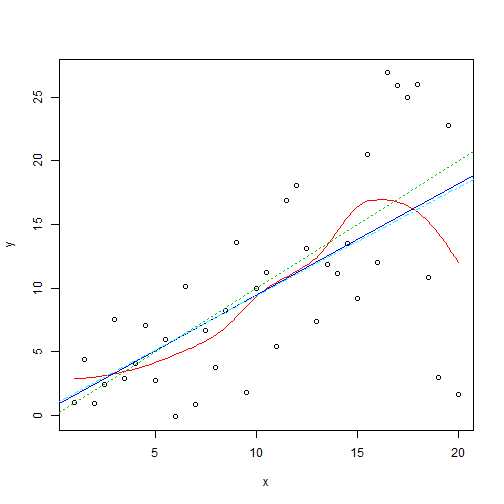``````
# A standard regression diagnostic plot to check for heteroscedasticity,
# that is, for unequal variances. The data are generated from a
# heteroscedastic process, so can you see this from this plot?
plot(fitted(fm), resid(fm), xlab = "Fitted Values", ylab = "Residuals")
``````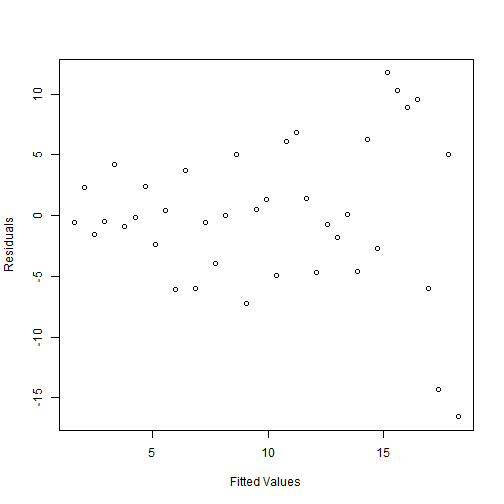``````
# A normal scores plot to check for skewness, kurtosis and outliers. (Note
# that the heteroscedasticity may show as apparentnon-normality.)
qqnorm(resid(fm))
qqline(resid(fm))
``````